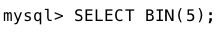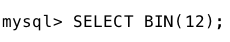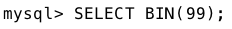# MySQL | BIN() Function

The BIN() function in MySQL is used to convert a decimal number to its binary equivalent.The BIN() function is equivalent to the CONV() function written in the format CONV(number,10,2). In this format CONV() function converts the number ‘number’ from base 10(decimal) to base 2(binary).

It is important to note that the BIN() function can only be used with positive integers. If you try to use a negative number or a non-integer value as the input to the BIN() function, MySQL will return an error.

Syntax :

```BIN(decimal_number)
```

Parameters Used: Here, decimal_number is the decimal number that you want to convert to binary.  Return Value: The BIN() function returns a string value, the binary equivalent of the decimal number passed as argument. It returns NULL if the number is NULL.

Examples:

1. Converting 5 to its binary equivalent. Syntax:Output:
`'101'`
1.
2. Converting 12 to its binary equivalent. Syntax:Output:
`'1100'`
1.
2. Converting 97 to its binary equivalent. Syntax:Output:
`'1100001'`
1.
Unlock the Power of Placement Preparation!
Feeling lost in OS, DBMS, CN, SQL, and DSA chaos? Our Complete Interview Preparation Course is the ultimate guide to conquer placements. Trusted by over 100,000+ geeks, this course is your roadmap to interview triumph.